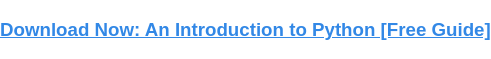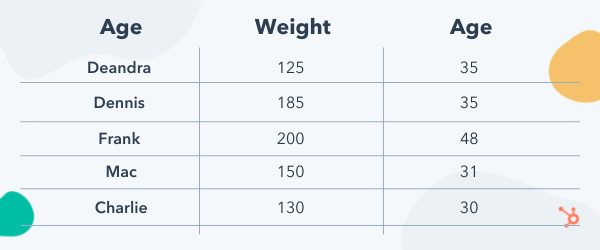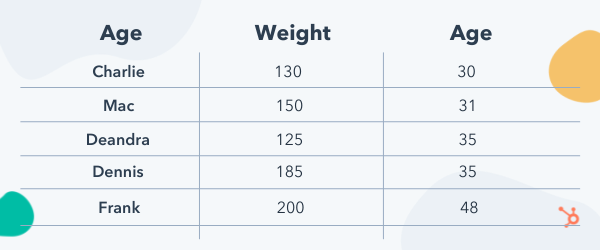# An Introduction to Pandas Dataframe SortingPublished:

Pandas dataframes are two-dimensional, labeled data structures containing data columns compatible with varying data types. It is very similar to spreadsheets, SQL tables, and dictionaries of Series objects. Furthermore, the dataframe is one of the most commonly used pandas objects and has many ways to interact with it.This post will cover one of the ways to interact with a dataframe object called sorting. You will learn the basics of dataframe sorting, how to use it, and its benefits. You will also learn how to sort dataframe columns and become familiar with the process.## What is pandas dataframe sorting?

Pandas dataframe sorting is a necessary skill to understand, as sorting and organizing data will always be an essential task. Data must be managed regularly in any programming field, and much of it can be automated. However, specific data management tasks will require a human touch; this is where understanding dataframe sorting comes into play.

## Pandas Sort by Column

Sorting through columns assists in organizing data within a pandas data collection, stored in a dataframe. Sorting data within a dataframe can be accomplished using the sort_values() function; the default value of this function is to sort in ascending order.

However, you can pass an argument to the function that disables the default behavior, which will return the values in descending order. It is essential to note that the sort_values() function does not change the data within the pandas dataframe; instead, it returns an instance of the data in the new order.

Now that you understand how the sort function works let's look at a practical example.

## Pandas Sort Values

The default behavior of using the Python sort_values() function in pandas is to sort the targeted column in ascending order. In this post, we will focus primarily on how the sort_values() function works with columns as that is its primary purpose.

` `import pandas as pdsunny= {'name': ['Deandra', 'Dennis', 'Frank', 'Mac', 'Charlie'],'weight': [125, 185, 200, 150, 130],'age': [35, 35, 48, 31, 30]}df_marks = pd.DataFrame(sunny)``

You start by importing pandas as “pd,” then create a data structure consisting of keys that identify the columns you want. Then for each key, you assign a set of values that will populate each column.

After completing those steps, you will need to call the DataFrame method on the “pd” object passing in the data structure you created. This new dataframe should be named as you see fit, following the standard Python naming convention. In this case, the name follows the snake case naming convention — for obvious reasons.

The result of the above code would be a table that looks like the following.This table shows the results of creating the pandas dataframe with the code above; this is a neat and organized way to view data. However, if you want to see that data organized in an ascending or descending sequence, you’ll need to use the sort_values() function.

` `#sort dataframesorted_df = df_marks.sort_values(by='age')``

This line of code will sort the values in the age column in ascending order, and the following table shows the results.Sorting the column in descending order can be accomplished by simply adding an argument and passing in a value with it. Let’s see what that looks like next.

` `#sort dataframesorted_df = df_marks.sort_values(by='age', ascending=False)``

By passing in the ascendingargument with a value of False, the column will be sorted in descending order instead.

## Using the Python Pandas Sort Values Function

Working with data can be a little You have learned the basics of sorting pandas dataframe columns and how the sort values() function works. You are now armed and ready to work with the pandas dataframe columns and implement the sort_values() function into your development process.Topics: What Is Python?

## Related Articles

We're committed to your privacy. HubSpot uses the information you provide to us to contact you about our relevant content, products, and services. You may unsubscribe from these communications at any time. For more information, check out our Privacy Policy.

A guide for marketers, developers, and data analysts.